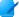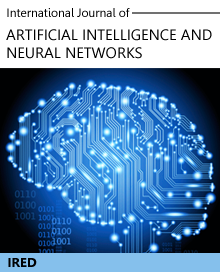# Journals Proceedings

International Journal of Artificial Intelligence and Neural Networks

#### A New Algorithm for Solving Fuzzy Constrained Shortest Path Problem using Intuitionistic Fuzzy Numbers

Author(s) : K. K. SHUKLA, MADHUSHI VERMAAbstract

Constrained shortest path problem (CSPP) is an NP-Complete problem where the goal is to determine the cheapest path bounded by a given delay constraint. This problem finds application in several fields like television and transportation networks, ATM circuit routing, multimedia applications etc. In these kinds of applications it is important to provide quality of service (QoS). Therefore, it is necessary to model the uncertainty involved in the parameters like cost, delay, time etc. The best technique to deal with the imprecise nature of the parameters is to represent them using fuzzy numbers. We propose a solution for the intuitionistic fuzzy version of the problem i.e. constrained intuitionistic fuzzy shortest path problem (CIFSPP) where one of the parameter i.e. cost is represented as a trapezoidal intuitionistic fuzzy number (TIFN) and the other parameter i.e. delay is modelled using real numbers. In this paper, we prefer intuitionistic fuzzy sets (IFS) over ordinary fuzzy set because using IFS both membership and non-membership as well as hesitancy degrees can be represented. Therefore, IFS provides a more realistic picture of the practical situation as the uncertainty can be analyzed in a better way using the degrees of belongingness and non-belongingness. One problem that exists in the fuzzy representation is ranking of the parameters since fuzzy numbers cannot be ordered naturally. We introduce a centroid based technique of ranking that is different from the existing methods as it uses an eight variable representation for TIFN and is a more generalized method of representing TIFNs. Therefore, it is more appropriate for problems like CIFSPP. An algorithm based on the TIFN ranking method is given and experimental results presented by applying it on large random graphs generated using a power law random graph generator gengraph-win. Also, we verify experimentally that the parameters in CIFSPP show the same behaviour as in the crisp version of the algorithm when the delay constraint is relaxed sufficiently.

No fo Author(s) : 2
Page(s) : 38 - 42
Electronic ISSN : 2250-3749
Volume 5 : Issue 1